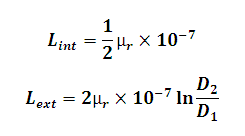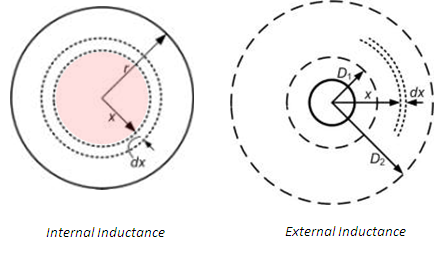# Line Inductance Calculator

This Calctown Calculator calculates the internal and external inductance of a current carrying solid line conductor.

Here µ0 = 4p * 10-7. Please enter the RELATIVE permeability accordingly.

unit
m
m

#### Result

H
HClick here to view image

where

Lint = internal inductance which is constant for all conductors at given μ

Lext = external inductance between external points distances D1 and D2 from the center of line

μr = relative permeability